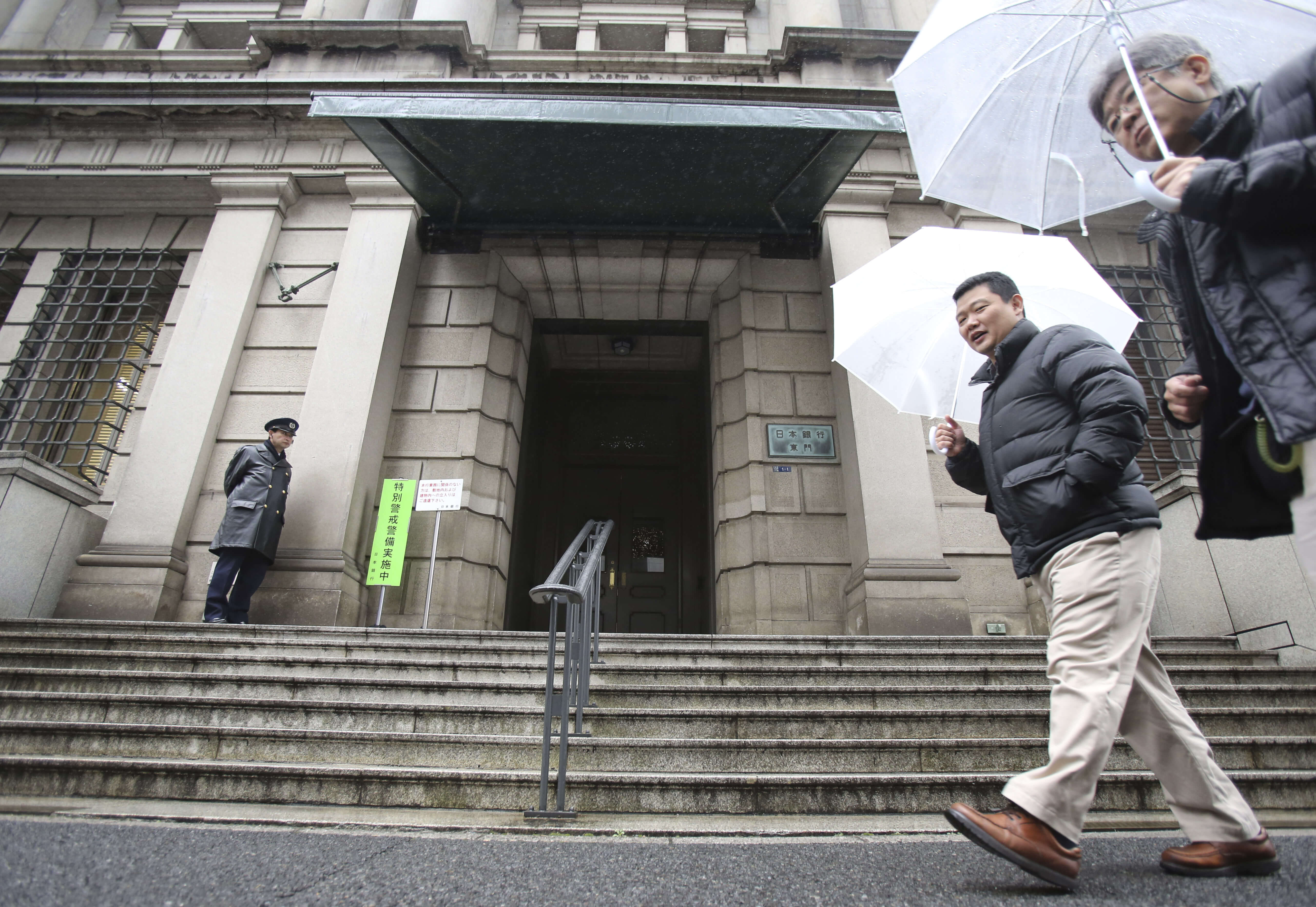# Cash [demand] rules everything around usOn Friday morning, the Bank of Japan broke a barrier that economists once thought was impervious: The central bank set its interest rate at negative 0.1 percent, below the “zero lower bound.”

The Bank of Japan isn’t the first central bank to enter this undiscovered country, though. The central banks of Denmark, Sweden, and Switzerland have also moved their rates below zero, and the European Central Bank has a negative rate on bank deposits. But Japan is the largest economy to go fully below zero as it tries to boost its long-ailing economy.

What’s more, the Bank of Japan has signaled that it’s prepared to push rates further negative. So how far can they push? And how much would they want to?

First, it’s worthwhile to work through why economists thought zero was a lower bound for interest rates in the first place. The answer has to do with how much people would demand cash once interest rates went negative. The belief was that if a central bank tried to set nominal interest rates below zero, then the population would shift their savings all into cash—which wouldn’t have a negative return—and would continue to demand cash. In other words, the demand for cash would be infinite.

But looking at the past few years, the demand for cash doesn’t appear to be infinite in the places with sub-zero interest rates. Now the question has shifted to the appropriate use of negative interest rates. That’s exactly the focus of a recent paper by Matthew Rognlie of the Massachusetts Institute of Technology.

In short, the effectiveness of negative interest rates depends on the demand for cash, and how that demand changes as interest rates go lower. Extremely high demand for cash, and the resulting glut of cash, can have negative impacts on the economy. Very high levels of cash demand with negative rates would mean the central bank is constantly losing money and would eventually need to be bailed out by taxpayers. At the same time, if too much money is being held as cash instead of as a bank deposit, that money can’t be lent out to borrowers—it’s just sitting under a mattress.

But the increase in aggregate demand for the economy spurred by the sub-zero interest rates can outweigh the negative aspects. It’s just a matter of how cash demand reacts. If demand for cash increases at a small rate as interest rates go lower (the interest rate elasticity of cash demand is low), then rates can go quite negative. But if the elasticity is high, then rates might not get very negative. The effective lower bound is then the interest rate where cash demand becomes infinite.

Rognlie’s model has interesting ramifications for one idea for that deals with the zero lower bound: eliminating cash. Rognlie points out that this idea makes more sense in a world where cash demand is very high and elastic. But if demand is low and inelastic, then interest rates can go negative without having to abolish cash. He also points out that there might be more intermediate ways to reform cash to reduce demand for it—the elimination of high-denomination bills, for example. When interest rates get lower, the demand for \$100 bills will increase relatively more than the demand for \$1 bills. By getting rid of \$100 bills, you’d reduce cash demand and allow interest rates to go lower.

Storage costs are another way that reduce demand for higher denomination bills. Many people have tried to determine the feasible lower bound by looking at the storage cost of cash. If storage costs are higher, then banks can charge depositors more and the interest rate can go lower. As you can see in the table at the top of this piece by David Keohane from FT Alphaville, the lower the highest denomination of a currency, the more space the currency takes up. If the only form of cash is, say, \$1 bills, then you’ll need a whole lot more storage space to store the same amount of money. So that reduces the demand for cash and further decreases the lower bound.

Rognlie’s focus on cash demand is illuminating but can only get us so far. The problem is that we don’t yet have good estimates of cash demand at negative interest rates. It’s something for the economics profession to figure out, but at least we now have a better idea of what to focus on.

Nick Bunker

#### Topics

Connect with us!

Explore the Equitable Growth network of experts around the country and get answers to today's most pressing questions!